## RD Sharma Class 9 Solutions Chapter 1 Number Systems Ex 1.5

These Solutions are part of RD Sharma Class 9 Solutions. Here we have given RD Sharma Class 9 Solutions Chapter 1 Number Systems Ex 1.5

Question 1.
Complete the following sentences:
(i) Every point on the number line corresponds to a … number which many be either … or
(ii) The decimal form of an irrational number is neither … nor …
(iii) The decimal representation of a rational number is either … or …
(iv) Every real number is either … number or … number.
Solution:
(i) Every point on the number line corresponds to a real number which many be either rational or irrational.
(ii) The decimal form of an irrational number is neither terminating nor repeating.
(iii) The decimal representation of a rational number is either terminating or non­terminating, recurring.
(iv) Every real number is either rational number or an irrational number.

Question 2.
Find whether the following statements are true or false:
(i)  Every real number is either rational or irrational.
(ii) π is an irrational number.
(iii) Irrational numbers cannot be represented by points on the number line.
Solution:
(i) True. (Value of π = 3.14)
(ii) False : we can represent irrational number also.

Question 3.
Represent $$\sqrt { 6 }$$, $$\sqrt { 7 }$$, $$\sqrt { 8 }$$ on the number line.
Solution: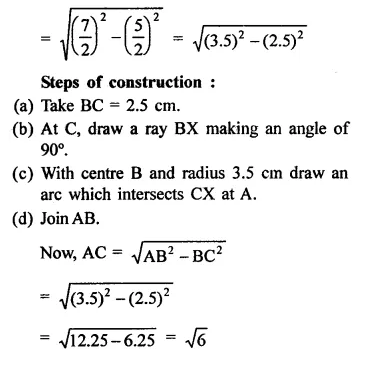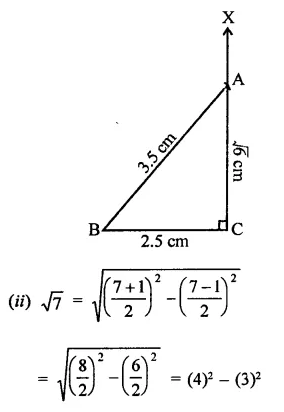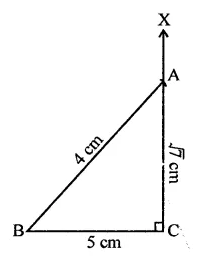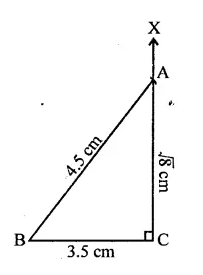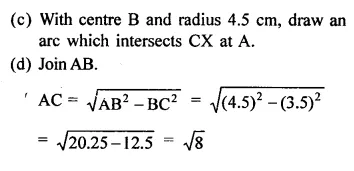Question 4.
Represent $$\sqrt { 3.5 }$$ , $$\sqrt { 9.4 }$$and $$\sqrt { 10.5 }$$ on the real number line.
Solution: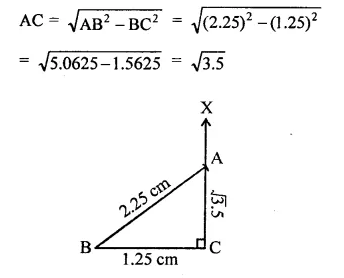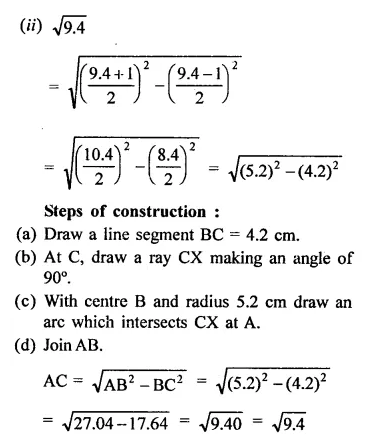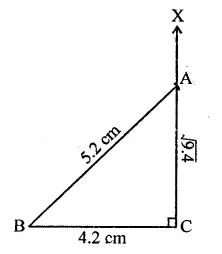Hope given RD Sharma Class 9 Solutions Chapter 1 Number Systems Ex 1.5 are helpful to complete your math homework.

If you have any doubts, please comment below. Learn Insta try to provide online math tutoring for you.

## RD Sharma Class 9 Solutions Chapter 1 Number Systems Ex 1.4

These Solutions are part of RD Sharma Class 9 Solutions. Here we have given RD Sharma Class 9 Solutions Chapter 1 Number Systems Ex 1.4

Question 1.
Define an irrational number.
Solution:
A number which cannot be expressed in the form of $$\frac { p }{ q }$$ where p and q are integers and q ≠ 0 is called an irrational number.

Question 2.
Explain, how irrational numbers differ from rational numbers?
Solution:
A rational number can be expressed in either terminating decimal or non-terminating recurring decimals but an irrational number is expressed in non-terminating non-recurring decimals.

Question 3.
Examine, whether the following numbers are rational or irrational: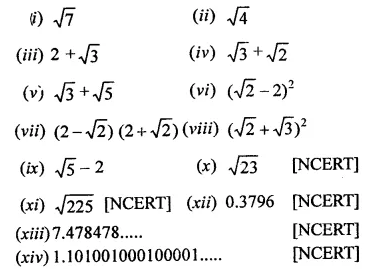Solution: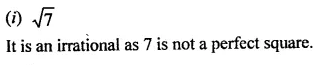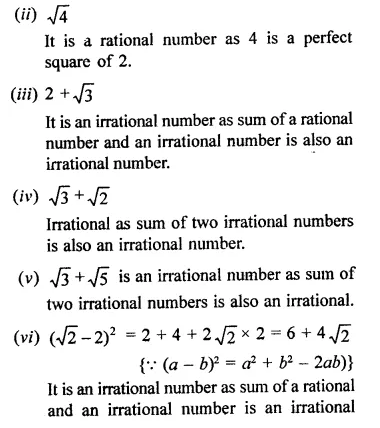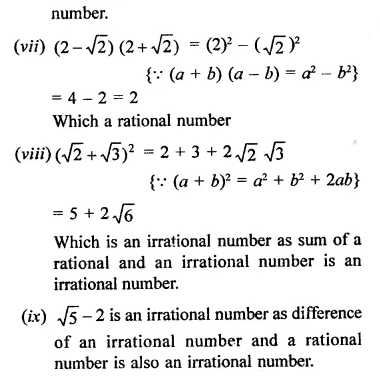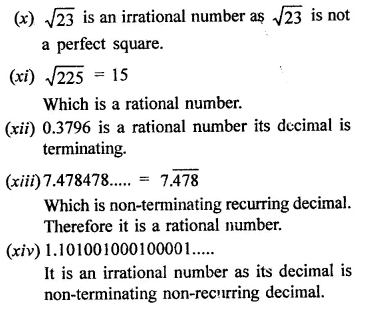Question 4.
Identify the following as rational or irrational numbers. Give the decimal representation of rational numbers: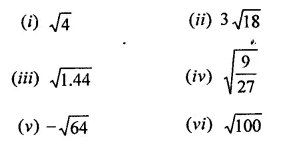Solution: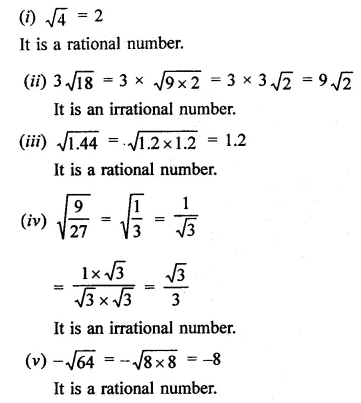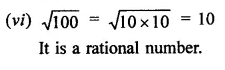Question 5.
In  the following equation, find which variables x, y, z etc. represent rational or irrational numbers: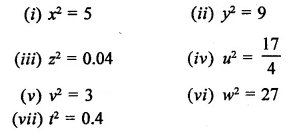Solution: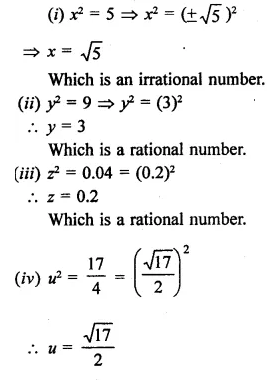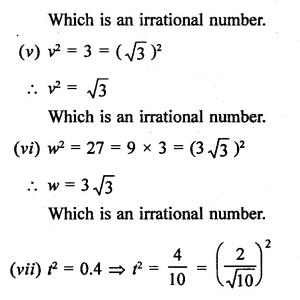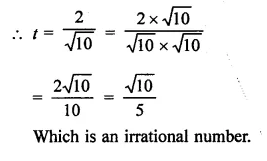Question 6.
Given two rational numbers lying between 0.232332333233332… and 0.212112111211112.
Solution:
Two rational numbers lying between 0.232332333233332… and 0.212112111211112… will be 0.232 and 0.212

Question 7.
Give two rational numbers lying between 0.515115111511115… and 0.5353353335…
Solution:
Two rational numbers lying between 0.515115111511115… and 0.535335333533335… will be 0.515, 0.535

Question 8.
Find one irrational numbers between 0.2101 and 0.2222… = 0.$$\overline { 2 }$$.
Solution:
One irrational number lying between 0.2101 and 0.2222… = 0.$$\overline { 2 }$$ will be 2201.0010001…

Question 9.
Find a rational number and also an irrational number lying between the numbers, 0.3030030003… and 0.3010010001…
Solution:
Between two numbers 0.3030030003… and 0.3010010001…, a rational will be 0.301 and irrational number will be 0.3020020002…

Question 10.
Find three different irrational numbers between the rational numbers $$\frac { 5 }{ 7 }$$ and $$\frac { 9 }{ 11 }$$. [NCERT]
Solution: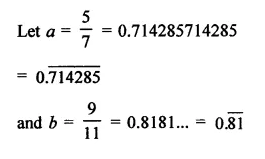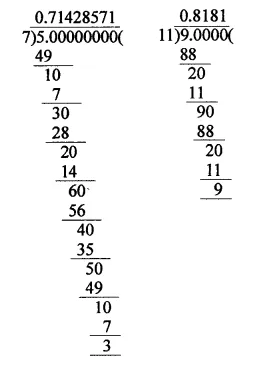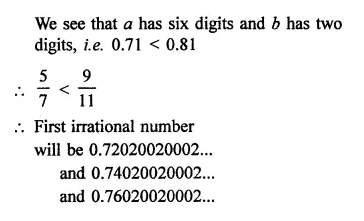Question 11.
Give an example of each, of two irrational numbers whose:
(i) difference is a rational number.
(ii) difference is an irrational number.
(iii) sum is a rational number.
(iv) sum is an irrational number.
(v) product is a rational number.
(vi) product is an irrational number.
(vii) quotient is a rational number.
(viii) quotient is an irrational number.
Solution:
(i) Two numbers whose difference is also a rational number, e.g. $$\sqrt { 2 }$$
, $$\sqrt { 2 }$$  which are irrational numbers.
∴ Difference = $$\sqrt { 2 }$$ – $$\sqrt { 2 }$$ = 0 which is also a rational number.
(ii) Two numbers whose difference is an irrational number.
e.g. $$\sqrt { 3 }$$  and $$\sqrt { 2 }$$  which are irrational numbers.
Now difference = $$\sqrt { 3 }$$  –$$\sqrt { 2 }$$  which is also an irrational number.
(iii) Let two irrational numbers be $$\sqrt { 3 }$$  and –$$\sqrt { 2 }$$  which are irrational numbers.
Now sum = $$\sqrt { 3 }$$  + (-$$\sqrt { 3 }$$) = $$\sqrt { 3 }$$
– $$\sqrt { 3 }$$  = 0 Which is a rational number.
(iv) Let two numbers be $$\sqrt { 5 }$$  , $$\sqrt { 3 }$$ which are irrational numbers.
Now sum = $$\sqrt { 5 }$$ + $$\sqrt { 3 }$$  which is an irrational number.
(v) Let numbers be $$\sqrt { 3 }$$  +$$\sqrt { 2 }$$and  $$\sqrt { 3 }$$  –$$\sqrt { 2 }$$which are irrational numbers.
Now product = ($$\sqrt { 3}$$  +$$\sqrt { 2 }$$ ) ($$\sqrt { 3 }$$ –$$\sqrt { 2 }$$)
= 3-2 = 1 which is a rational number.
(vi) Let numbers be $$\sqrt { 3 }$$ and $$\sqrt { 5 }$$ , which are irrational number.
Now product = $$\sqrt { 3 }$$ x $$\sqrt { 5 }$$  = $$\sqrt { 3×5 }$$
= $$\sqrt { 15 }$$
which is an irrational number.
(vii) Let numbers be 6 $$\sqrt { 2 }$$  and 2 $$\sqrt { 2 }$$ which are irrational numbers.
Quotient =$$\frac { 6\sqrt { 2 } }{ 2\sqrt { 2 } }$$ = 3 which is a rational number.
(viii) Let numbers be $$\sqrt { 3 }$$and $$\sqrt { 5 }$$ which are irrational numbers.
Now quotient =$$\frac { \sqrt { 3 } }{ \sqrt { 5 } }$$ = $$\sqrt { \frac { 3 }{ 5 } }$$ which is an  irrational number.

Question 12.
Find two irrational numbers between 0.5 and 0.55.
Solution:
Two irrational numbers between 0.5 and 0.55 will be 0.51010010001… and 52020020002…

Question 13.
Find two irrational numbers lying betwee 0.1 and 0.12.
Solution:
Two irrational numbers lying between 0.1 and 0.12 will be 0.1010010001… and 0.1020020002…

Question 14.
Prove that $$\sqrt { 3 }$$+$$\sqrt { 5 }$$ is an irrational number.
Solution: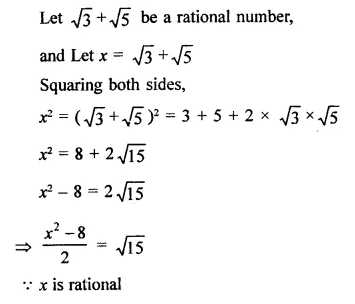Hope given RD Sharma Class 9 Solutions Chapter 1 Number Systems Ex 1.4 are helpful to complete your math homework.

If you have any doubts, please comment below. Learn Insta try to provide online math tutoring for you.

## RD Sharma Class 9 Solutions Chapter 1 Number Systems Ex 1.3

These Solutions are part of RD Sharma Class 9 Solutions. Here we have given RD Sharma Class 9 Solutions Chapter 1 Number Systems Ex 1.3

Question 1.
Express each of the following decimals in the form $$\frac { p }{ q }$$:
(i) 0.39
(ii) 0.750
(iii) 2.15
(iv) 7.010
(v) 9.90
(vi) 1.0001
Solution:Question 2.
Express each of the following decimals in the form $$\frac { p }{ q }$$: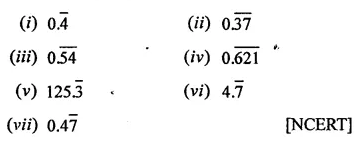Solution: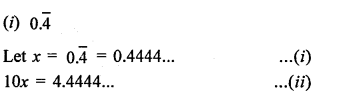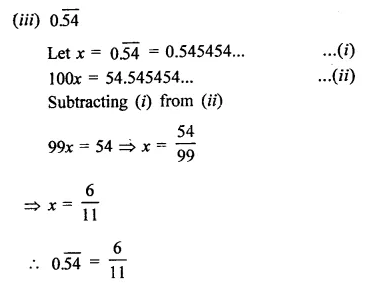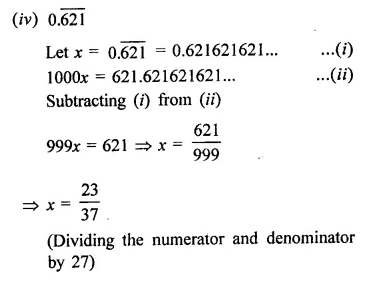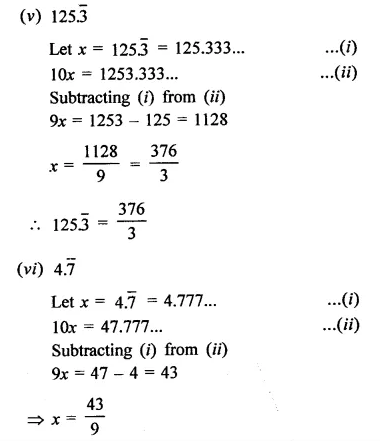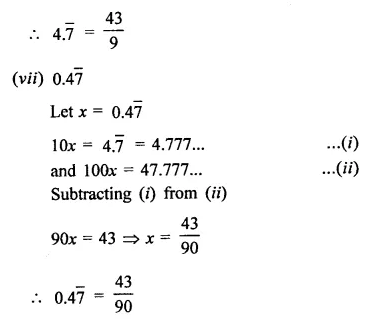Hope given RD Sharma Class 9 Solutions Chapter 1 Number Systems Ex 1.3 are helpful to complete your math homework.

If you have any doubts, please comment below. Learn Insta try to provide online math tutoring for you.

## RD Sharma Class 9 Solutions Chapter 1 Number Systems Ex 1.2

These Solutions are part of RD Sharma Class 9 Solutions. Here we have given RD Sharma Class 9 Solutions Chapter 1 Number Systems Ex 1.2

Question 1.
Express the following rational numbers as decimals: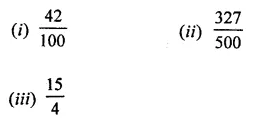Solution:Question 2.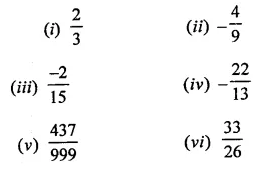Solution: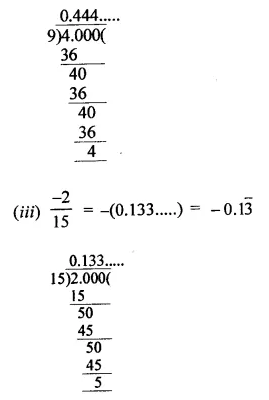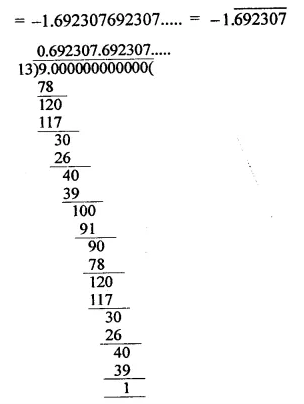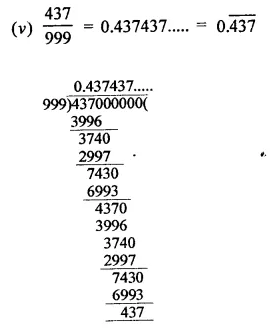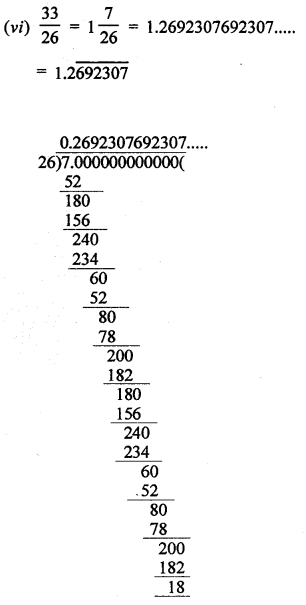Question 3.
Look at several examples of rational numbers in the form $$\frac { p }{ q }$$ (q ≠ 0), where p and q are integers with no common factors other than 1 and having terminating decimal representations. Can you guess what property q must satisfy?
Solution:
The property is if the denominators have factors 2 or 5 or both, the decimal representation will be terminating e.g.Hope given RD Sharma Class 9 Solutions Chapter 1 Number Systems Ex 1.2 are helpful to complete your math homework.

If you have any doubts, please comment below. Learn Insta try to provide online math tutoring for you.

## RD Sharma Class 9 Solutions Chapter 1 Number Systems Ex 1.1

These Solutions are part of RD Sharma Class 9 Solutions. Here we have given RD Sharma Class 9 Solutions Chapter 1 Number Systems Ex 1.1

Question 1.
Is zero a rational number? Can you write it P in the form $$\frac { p }{ q }$$ , where p and q are integers and q ≠ 0? [NCERT]
Solution:
Yes, zero is a rational number e.g.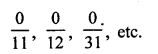Question 2.
Find five rational numbers between 1 and 2. [NCERT]
Solution:
We know that one rational number between two numbers a and b = $$\frac { a+b }{ 2 }$$
Therefore one rational number between 1 and 2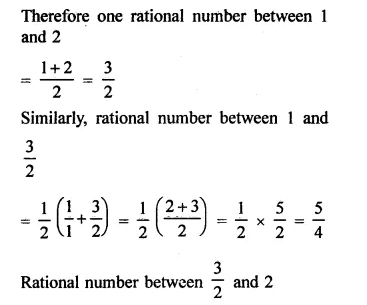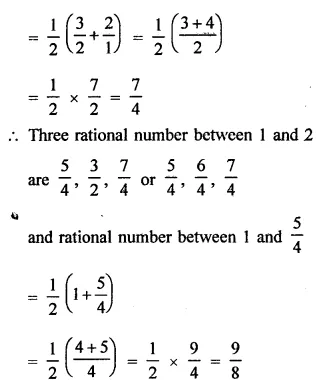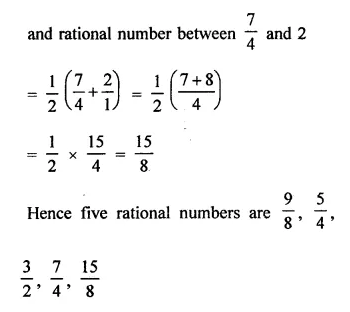Question 3.
Find six rational numbers between 3 and 4. [NCERT]
Solution:
One rational number between 3 and 4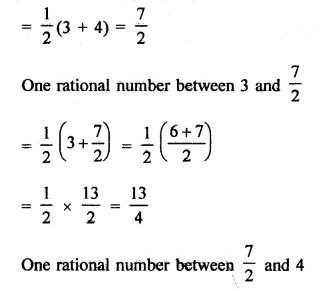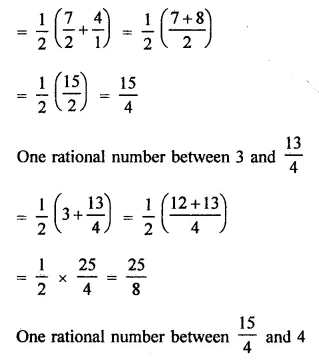Question 4.
Find five rational numbers between $$\frac { 3 }{ 5 }$$ and $$\frac { 4 }{ 5 }$$
Solution: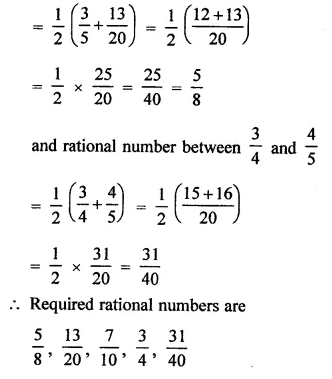Question 5.
Are the following statements true or false?
(i) Every whole number is a natural number. [NCERT]
(ii) Every integer is a rational number.
(iii) Every rational number is an integer.
(iv) Every natural number is a whole number,
(v) Every integer is a whole number.
(vi) Every rational number is a whole number.
Solution:
(i) False, as 0 is not a natural number.
(ii) True.
(iii) False, as $$\frac { 1 }{ 2 }$$, $$\frac { 1 }{ 3 }$$ etc. are not integers.
(iv) True.
(v) False, ∵ negative natural numbers are not whole numbers.
(vi) False, ∵ proper fraction are not whole numbers

Hope given RD Sharma Class 9 Solutions Chapter 1 Number Systems Ex 1.1 are helpful to complete your math homework.

If you have any doubts, please comment below. Learn Insta try to provide online math tutoring for you.

## Online Education for RD Sharma Class 9 Solutions Chapter 15 Areas of Parallelograms and Triangles MCQS

These Solutions are part of Online Education RD Sharma Class 9 Solutions. Here we have given RD Sharma Class 9 Solutions Chapter 15 Areas of Parallelograms and Triangles MCQS

Other Exercises

Mark the correct alternative in each of the following:
Question 1.
If the length of a chord of a circle is 16 cm and is at a distance of 15 cm from the centre of the circle, then the radius of the circle is
(a) 15 cm
(b) 16 cm
(c) 17 cm
(d) 34 cm
Solution:
Length of chord AB of circle = 16 cm
Distance from the centre OL = 15 cm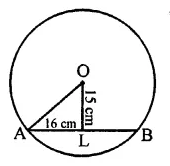Let OA be the radius, then in right ∆OAL,
OA2 = OL2 + AL2
16
= (15)2 + $$\frac { 16 }{ 2 }$$ = 152 + 82
= 225 + 64 = 289 = (17)2
∴ OA = 17 cm
Hence radius of the circle = 17 cm (c)

Question 2.
The radius of a circle Js 6 cm. The perpendicular distance from the centre of the circle to the chord which is 8 cm in length, isSolution:
Radius of the cirlce (r) = 6 cm
Perpendicular distance from centre = ?
Length of chord = 8 cm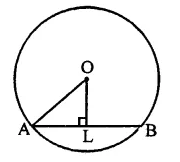Let AB be chord, OL is the distance
In right ∆OAL
OA2 = AL2 + OL2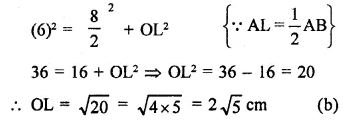Question 3.
If O is the centre of a circle of radius r and AB is a chord of the circle at a distance $$\frac { r }{ 2 }$$ from O, then ∠BAO =
(a) 60°
(b) 45°
(c) 30°
(d) 15°
Solution:
r is the radius of the circle with centre O
AB is the chord, at a distance of $$\frac { r }{ 2 }$$ from the centre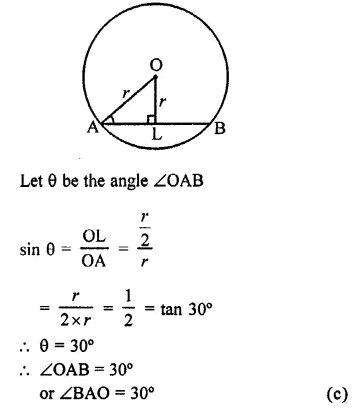Question 4.
ABCD is a cyclic quadrilateral such that ∠ADB = 30° and ∠DCA = 80°, then ∠DAB=
(a) 70°
(b) 100°
(c) 125°
(d) 150°
Solution:
ABCD is a cyclic quadrilateral ∠DCA = 80° and ∠ADB = 30°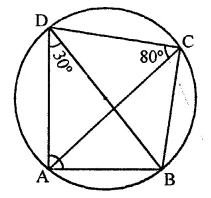∵∠ADB = ∠ACB (Angles in the same segment)
∴ ∠ACB = 30°
∴ ∠BCD = 80° + 30° = 110°
∵ ABCD is a cyclic quadrilateral
⇒ ∠BAD = 180°- 110° = 70°
or ∠DAB = 70° (a)

Question 5.
A chord of length 14 cm is at a distance of 6 cm from the centre of a circle. The length of another chord at a distance of 2 cm from the centre of the circle is
(a) 12 cm
(b) 14 cm
(c) 16 cm
(d) 18 cm
Solution:
In a circle AB chord = 14 cm
and distance from centre OL = 6 cm
Let r be the radius of the circle, then OA2 = AL2 + OL2
⇒ r2 = (7)2 + (6)2 = 49 + 36 = 85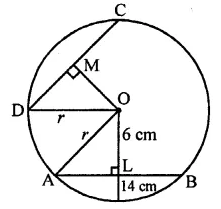In the same circle length of another chord CD = ?
Distance from centre = 2 cm
∴ r2 = OM2 + MD2
⇒ 85 = (2)2 + DM2
⇒ 85 = 4 + DM2
⇒ DM2 = 85-4 = 81 = (9)2
∴ DM = 9
∴ CD = 2 x DM = 2 x 9 = 18 cm
∴Length of another chord = 18 cm (d)

Question 6.
One chord of a circle is known to be 10 cm. The radius of this circle must be
(a) 5 cm
(b) greater than 5 cm
(c) greater than or equal to 5 cm
(d) less than 5 cm
Solution:
Length of chord of a circle = 10 cm
Length of radius of the circle greater than half of the chord
More than $$\frac { 10 }{ 2 }$$ = 5 cm (b)

Question 7.
ABC is a triangle with B as right angle, AC = 5 cm and AB = 4 cm. A circle is drawn with O as centre and OC as radius. The length of the chord of this circle passing through C and B is
(a) 3 cm
(b) 4 cm
(c) 5 cm
(d) 6 cm
Solution:
In right ∆ABC, ∠B = 90°
AC = 5 cm, AB = 4 cm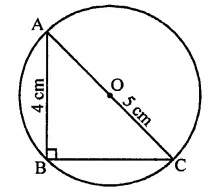∴ BC2 = AC-AB2
= 52 – 42 = 25 – 16
= 9 = (3)2
∴ BC = 3 cm
∴ Length of chord BC = 3 cm (a)

Question 8.
If AB, BC and CD are equal chords of a circle with O as centre, and AD diameter then ∠AOB =
(a) 60°
(b) 90°
(c) 120°
(d) none of these
Solution:
In a circle chords AB = BC = CD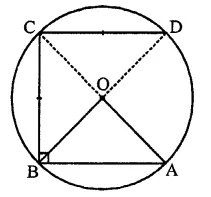O is the centre of the circle
∴ ∠AOB = cannot be found (d)

Question 9.
Let C be the mid-point of an arc AB of a circle such that m $$\breve { AB }$$ = 183°. If the region bounded by the arc ACB and line segment AB is denoted by S, then the centre O of the circle lies
(a) in the interior of S
(b) in the exterior of S
(c) on the segment AB
(d) on AB and bisects AB
Solution:
$$\breve { AB }$$ = 183°
∴ AB is the diameter of the circle with centre O and C is the mid point of arc AB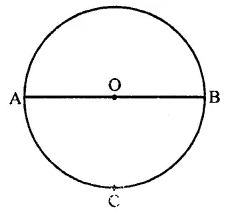Line segment AB = S
∴ Centre will lie on AB (c)

Question 10.
In a circle, the major arc is 3 ti.nes the minor arc. The corresponding central angles and the degree measures of two arcs are
(a) 90° and 270°
(b) 90° and 90°
(d) 60° and 210°
Solution:
In a circle, major arc is 3 times the minor arc i.e. arc ACB = 3 arc ADB
∴ Reflex ∠AOB = 3∠AOB
But angle at O = 360°and let ∠AOB = x
x + 3x – 360°
⇒ 4x = 360°
⇒ x = $$\frac { { 62 }^{ \circ } }{ 2 }$$  = 90°
∴ 3x = 90° x 3 = 270°
Here angles are 270° and 90° (c)

Question 11.
If A and B are two points on a circle such that m($$\breve { AB }$$) = 260°. A possible value for the angle subtended by arc BA at a point on the circle is
(a) 100°
(b) 75°
(c) 50°
(d) 25°
Solution:
A and B are two points on the circle such that reflex ∠AOB = 260°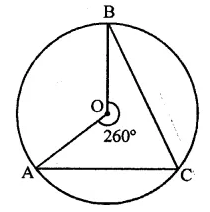∴ ∠AOB = 360° – 260° = 100°
C is a point on the circle
∴ By joining AC and BC,
∠ACB = $$\frac { 1 }{ 2 }$$∠AOB = $$\frac { 1 }{ 2 }$$ x 100° = 50° (c)

Question 12.
An equilateral triangle ABC is inscribed in a circle with centre O. The measures of ∠BOC is
(a) 30°
(b) 60°
(c) 90°
(d) 120°
Solution:
∆ABC is an equilateral triangle inscribed in a circle with centre O∴ Measure of ∠BOC = 2∠BAC
= 2 x 60° = 120° (d)

Question 13.
If two diameters of a circle intersect each other at right angles, then quadrilateral formed by joining their end points is a
(a) rhombus
(b) rectangle
(c) parallelogram
(d) square
Solution:
Two diameter of a circle AB and CD intersect each other at right angles∵ The diagonals are equal and bisect each other at right angles
∴ ACBD is a square (d)

Question 14.
In ABC is an arc of a circle and ∠ABC = 135°, then the ratio of arc $$\breve { AB }$$ to the circumference is
(a) 1 : 4
(b) 3 : 4
(c) 3 : 8
(d) 1 : 2
Solution:
Arc ABC of a circle and ∠ABC = 135°
Join OA and OC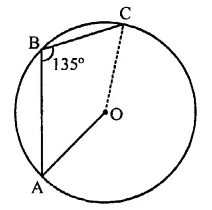∴ Angle subtended by arc ABC at the centre = 2 x ∠ABC = 2 x 135° = 270°
Angle at the centre of the circle = 360°
∴ Ratio with circumference = 270° : 360° = 3:4 (b)

Question 15.
The chord of a circle is equal to its radius. The angle subtended by this chord at the minor arc of the circle is
(a) 60°
(b) 75°
(c) 120°
(d) 150°
Solution:
The chord of a circle = radius of the circle In the figure OA = OB = AB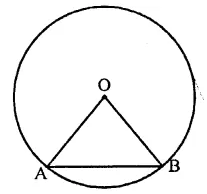∴ ∠AOB = 60°
(Each angle of an equilateral = 60°) (a)

Question 16.
PQRS is a cyclic quadrilateral such that PR is a diameter of the circle. If ∠QPR = 67° and ∠SPR = 72°, then ∠QRS =
(a) 41°
(b) 23°
(c) 67°
(d) 18°
Solution:
PQRS is a cyclic quadrilateral with centre O and ∠QPR = 67°
∠SPR = 72°∴ ∠QPS = 67° + 72° = 139°
∵ ∠QPS + ∠QRS = 180° (Sum of opposite angles of a cyclic quad.)
⇒ 139° + ∠QRS = 180°
⇒ ∠QRS = 180° – 139° = 41° (a)

Question 17.
If A, B, C are three points on a circle with centre O such that ∠AOB = 90° and ∠BOC = 120°, then ∠ABC =
(a) 60°
(b) 75°
(c) 90°
(d) 135°
Solution:
A, B and C are three points on a circle with centre O
∠AOB = 90° and ∠BOC = 120°∴ ∠AOC = 360° – (120° + 90°)
= 360° -210°= 150°
But ∠AOC is at the centre made by arc AC and ∠ABC at the remaining part of the circle
∴ ∠ABC = $$\frac { 1 }{ 2 }$$ ∠AOC
= $$\frac { 1 }{ 2 }$$ x 150° = 75° (b)

Question 18.
The greatest chord of a circle is called its
(b) secant
(c) diameter
(d) none of these
Solution:
The greatest chord of a circle is called its diameter. (c)

Question 19.
Angle formed in minor segment of a circle is
(a) acute
(b) obtuse
(c) right angle
(d) none of these
Solution:
The angle formed in minor segment of a circle is obtuse angle. (b)

Question 20.
Number of circles that can be drawn through three non-collinear points is
(a) 1
(b) 0
(c) 2
(d) 3
Solution:
The number of circles that can pass through three non-collinear points is only one. (a)

Question 21.
In the figure, if chords AB and CD of the circle intersect each other at right angles, then x + y =
(a) 45°
(b) 60°
(c) 75°
(d) 90°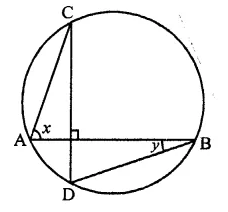Solution:
In the circle, AB and CD are two chords which intersect each other at P at right angle i.e. ∠CPB = 90°∠CAB and ∠CDB are in the same segment
∴ ∠CDB = ∠CAB = x
Now in ∆PDB,
Ext. ∠CPB = ∠D + ∠DBP
⇒ 90° = x + y (∵ CD ⊥ AB)
Hence x + y = 90° (d)

Question 22.
In the figure, if ∠ABC = 45°, then ∠AOC=
(a) 45°
(b) 60°
(c) 75°
(d) 90°Solution:
∵ arc AC subtends
∠AOC at the centre of the circle and ∠ABC
at the remaining part of the circle∴ ∠AOC = 2∠ABC
= 2 x 45° = 90°
Hence ∠AOC = 90° (d)

Question 23.
In the figure, chords AD and BC intersect each other at right angles at a point P. If ∠D AB = 35°, then ∠ADC =
(a) 35°
(b) 45°
(c) 55°
(d) 65°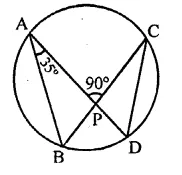Solution:
Two chords AD and BC intersect each other at right angles at P, ∠DAB = 35°
AB and CD are joined
In ∆ABP,
Ext. ∠APC = ∠B + ∠A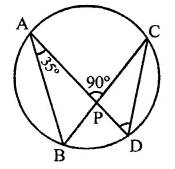⇒ 90° = ∠B + 35°
∠B = 90° – 35° = 55°
∵ ∠ABC and ∠ADC are in the same segment
∴ ∠ADC = ∠ABC = 55° (c)

Question 24.
In the figure, O is the centre of the circle and ∠BDC = 42°. The measure of ∠ACB is
(a) 42°
(b) 48°
(c) 58°
(d) 52°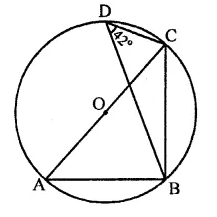Solution:
In the figure, O is the centre of the circle
∠BDC = 42°
∠ABC = 90° (Angle in a semicircle)
and ∠BAC and ∠BDC are in the same segment of the circle.
∴ ∠BAC = ∠BDC = 42°
Now in ∆ABC,
∠A + ∠ABC + ∠ACB = 180° (Sum of angles of a triangle)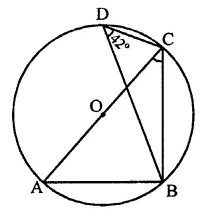⇒ 42° + 90° + ∠ACB = 180°
⇒ 132° + ∠ACB – 180°
⇒ ∠ACB = 180° – 132° = 48° (b)

Question 25.
In a circle with centre O, AB and CD are two diameters perpendicular to each other. The length of chord AC isSolution:
AB and CD are two diameters of a circle with centre O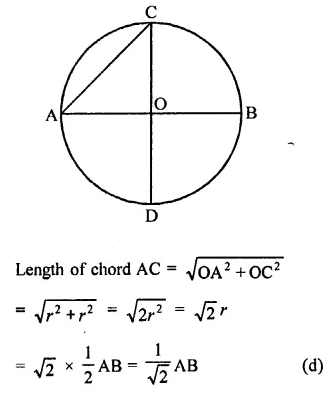Question 26.
Two equal circles of radius r intersect such that each passes through the centre of the other. The length of the common chord of the circles is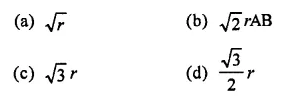Solution:
Two equal circles pass through the centre of the other and intersect each other at A and B
Let r be the radius of each circle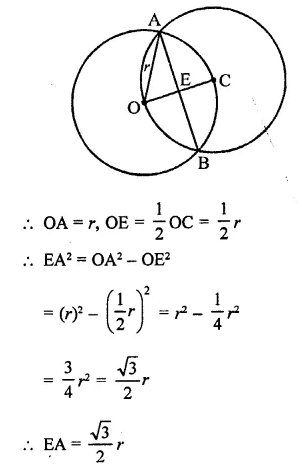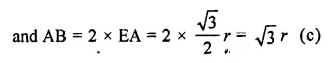Question 27.
If AB is a chord of a circle, P and Q are the two points on the circle different from A and B,then
(a) ∠APB = ∠AQB
(b) ∠APB + ∠AQB = 180° or ∠APB = ∠AQB
(c) ∠APB + ∠AQB = 90°
(d) ∠APB + ∠AQB = 180°
Solution:
AB is chord of a circle,
P and Q are two points other than from points A and B∵ ∠APB and ∠AQB are in the same segment of the circle
∴ ∠APB = ∠AQB (a)

Question 28.
AB and CD are two parallel chords of a circle with centre O such that AB = 6 cm and CD = 12 cm. The chords are on the same side of the centre and the distance between them is 3 cm. The radius of the circle isSolution:
AB and CD are two parallel chords of a circle with centre O
Let r be the radius of the circle AB = 6 cm, CD = 12 cm
and distance between them = 3 cm
Join OC and OA, LM = 3 cm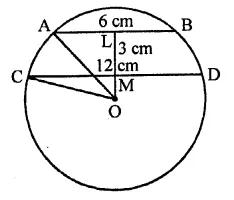Let OM = x, then OL = x + 3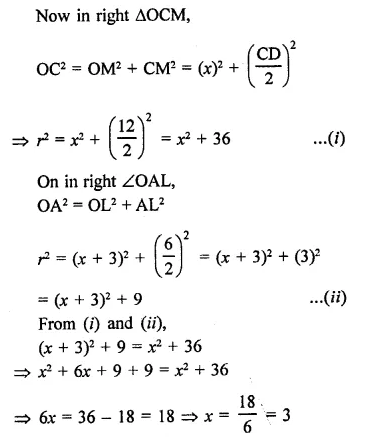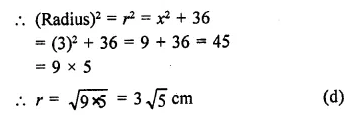Question 29.
In a circle of radius 17 cm, two parallel chords are drawn on opposite side of a diameter. This distance between the chords is 23 cm. If the length of one chord is 16 cm then the length of the other is
(a) 34 cm
(b) 15 cm
(c) 23 cm
(d) 30 cm
Solution:
Radius of a circle = 17 cm
The distance between two parallel chords = 23 cm
AB || CD and LM = 23 cm
Join OA and OC,
∴ OA = OC = 17 cm
Let OL = x, then OM = (23 – x) cm
AB = 16 cm
Now in right ∆OAL,
OA2 = OL22 + AL2
⇒ (17)2 = x2 + AL2
⇒ 289 = x2 + AL2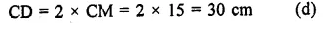Question 30.
In the figure, O is the centre of the circle such that ∠AOC = 130°, then ∠ABC =
(a) 130°
(b) 115°
(c) 65°
(d) 165°Solution:
O is the centre of the circle and ∠AOC = 130°
Reflex ∠AOC = 360° – 130° = 230°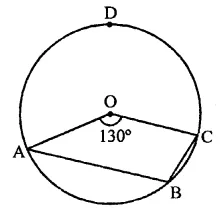Now arc ADB subtends ∠AOC at the centre and ∠ABC at the remaining part of the circle
∴ ∠ABC = $$\frac { 1 }{ 2 }$$reflex ∠AOC
= $$\frac { 1 }{ 2 }$$ x 230°= 115° (b)

Hope given RD Sharma Class 9 Solutions Chapter 15 Areas of Parallelograms and Triangles MCQS are helpful to complete your math homework.

If you have any doubts, please comment below. Learn Insta try to provide online math tutoring for you.